# Hypothesis Testing

Using computer simulation. Based on examples from the infer package. Code for Quiz 13.

Load the packages we will need

``````library(tidyverse)
library(infer)
library(skimr)
``````
• Replace all the instances of ???. These are answers on your moodle quiz.

• Run all the individual code chunks to make sure the answers in this file correspond with your quiz answers

• After you check all your code chunks run then you can knit it. It won’t knit until the ??? are replaced

• Save a plot to be your preview plot

# Question: t-test

• The data this quiz uses is a subset of HR

-Look at the variable definitions

• Note that the variables evaluation and salary have been recoded to be represented as words instead of numbers
• Set random seed generator to 123

``````set.seed(123)
``````

hr_2_tidy.csv is the name of your data subset

• Read it into and assign to hr

• Note: col_types = “fddfff” defines the column types factor-double-double-factor-factor-factor
``````hr  <- read_csv("https://estanny.com/static/week13/data/hr_2_tidy.csv",
col_types = "fddfff")
``````

use `skim` to summarize the data in `hr`

``````skim(hr)
``````
 Name hr Number of rows 500 Number of columns 6 _______________________ Column type frequency: factor 4 numeric 2 ________________________ Group variables None

Variable type: factor

skim_variable n_missing complete_rate ordered n_unique top_counts
gender 0 1 FALSE 2 mal: 256, fem: 244
evaluation 0 1 FALSE 4 bad: 154, fai: 142, goo: 108, ver: 96
salary 0 1 FALSE 6 lev: 95, lev: 94, lev: 87, lev: 85
status 0 1 FALSE 3 fir: 194, pro: 179, ok: 127

Variable type: numeric

skim_variable n_missing complete_rate mean sd p0 p25 p50 p75 p100 hist
age 0 1 39.86 11.55 20.3 29.60 40.2 50.1 59.9 ▇▇▇▇▇
hours 0 1 49.39 13.15 35.0 37.48 45.6 58.9 79.9 ▇▃▂▂▂

This means hours per week is: 49.4

## Q: Is the mean number of hours per week is 48?

`specify` that `hours` is the variable of interest

``````hr  %>%
specify(response = hours)
``````
``````Response: hours (numeric)
# A tibble: 500 × 1
hours
<dbl>
1  78.1
2  35.1
3  36.9
4  38.5
5  36.1
6  78.1
7  76
8  35.6
9  35.6
10  56.8
# … with 490 more rows``````

`hypothesize` that the average hours worked is 48

``````hr  %>%
specify(response = hours)  %>%
hypothesize(null = "point", mu = 48)
``````
``````Response: hours (numeric)
Null Hypothesis: point
# A tibble: 500 × 1
hours
<dbl>
1  78.1
2  35.1
3  36.9
4  38.5
5  36.1
6  78.1
7  76
8  35.6
9  35.6
10  56.8
# … with 490 more rows``````

`generate` 1000 replicates representing the null hypothesis

``````hr %>%
specify(response = hours)  %>%
hypothesize(null = "point", mu = 48)  %>%
generate(reps = 1000, type = "bootstrap")
``````
``````Response: hours (numeric)
Null Hypothesis: point
# A tibble: 500,000 × 2
# Groups:   replicate [1,000]
replicate hours
<int> <dbl>
1         1  39.7
2         1  44.3
3         1  46.8
4         1  33.7
5         1  39.6
6         1  39.5
7         1  40.5
8         1  55.8
9         1  72.6
10         1  35.7
# … with 499,990 more rows``````

The output has 500,000 rows

`calculate` the distribution of statistics from the generated data

• Assign the output `null_t_distribution`

• Display `null_t_distribution`

``````null_t_distribution  <- hr  %>%
specify(response = hours)  %>%
hypothesize(null = "point", mu = 48)  %>%
generate(reps = 1000, type = "bootstrap")  %>%
calculate(stat = "t")

null_t_distribution
``````
``````Response: hours (numeric)
Null Hypothesis: point
# A tibble: 1,000 × 2
replicate   stat
<int>  <dbl>
1         1  0.891
2         2 -0.526
3         3 -0.386
4         4 -0.893
5         5  0.491
6         6 -0.483
7         7  2.08
8         8 -1.23
9         9 -0.424
10        10 -1.21
# … with 990 more rows``````
• `null_t_distribution` has 1000 t-stats

`visualize` the simulated null distribution

``````visualize(null_t_distribution)
``````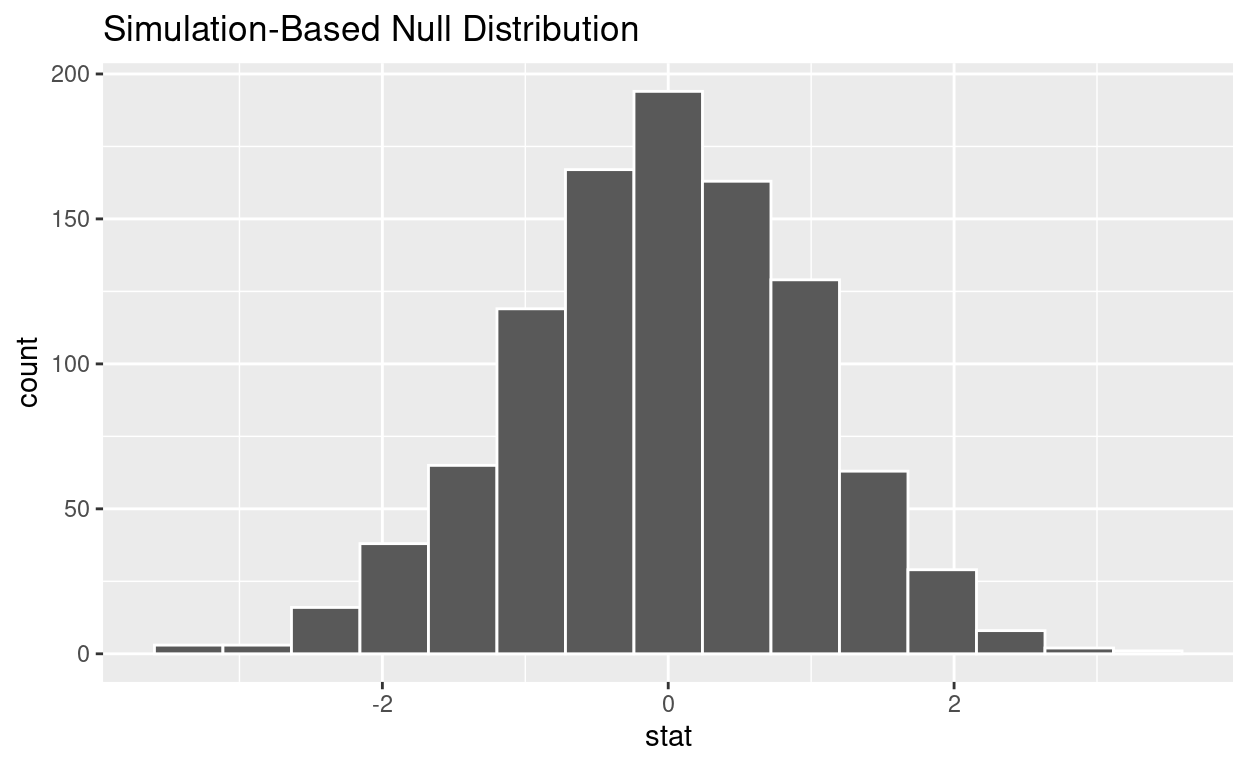`calculate` the statistic from your observed data

• Assign the output `observed_t_statistic`

• Display `observed_t_statistic`

``````observed_t_statistic  <- hr  %>%
specify(response = hours)  %>%
hypothesize(null = "point", mu = 48)  %>%
calculate(stat = "t")

observed_t_statistic
``````
``````Response: hours (numeric)
Null Hypothesis: point
# A tibble: 1 × 1
stat
<dbl>
1  2.37``````

get_p_value from the simulated null distribution and the observed statistic

``````null_t_distribution  %>%
get_p_value(obs_stat = observed_t_statistic, direction = "two-sided")
``````
``````# A tibble: 1 × 1
p_value
<dbl>
1   0.014``````

`shade_p_value` on the simulated null distribution

``````null_t_distribution  %>%
visualize() +
shade_p_value(obs_stat = observed_t_statistic, direction = "two-sided")
``````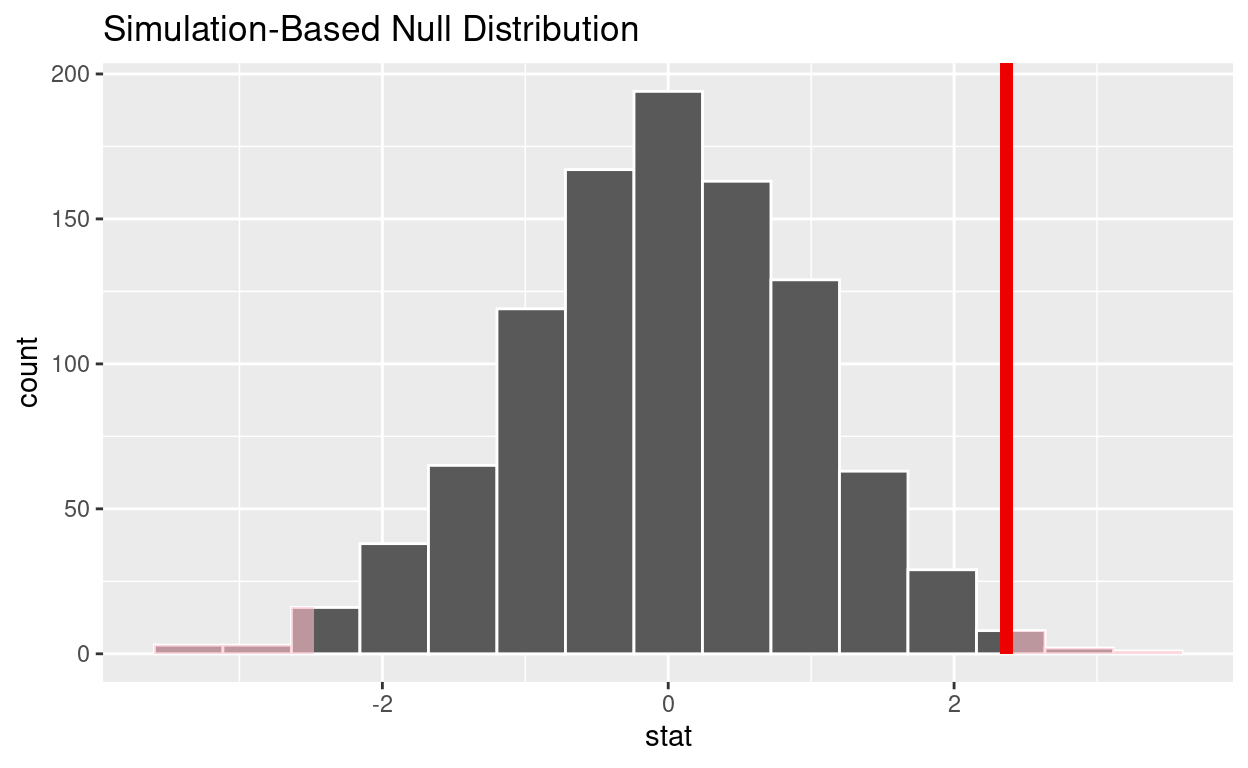Is the p-value < 0.05? yes

Does your analysis support the null hypothesis that the true mean number of hours worked was 48? no

# Question: 2 sample t-test

**hr_3_tidy.csv* *is the name of your data subset

• Read it into and assign to hr_2

• Note: col_types = “fddfff” defines the column types factor-double-double-factor-factor-factor

``````hr_2  <- read_csv("https://estanny.com/static/week13/data/hr_3_tidy.csv",
col_types = "fddfff")
``````

## Q: Is the average number of hours worked the same for both genders?

use `skim` to summarize the data in `hr_2` by `gender`

``````hr_2 %>%
group_by(gender)  %>%
skim()
``````
 Name Piped data Number of rows 500 Number of columns 6 _______________________ Column type frequency: factor 3 numeric 2 ________________________ Group variables gender

Variable type: factor

skim_variable gender n_missing complete_rate ordered n_unique top_counts
evaluation male 0 1 FALSE 4 bad: 72, fai: 67, goo: 61, ver: 47
evaluation female 0 1 FALSE 4 bad: 76, fai: 71, goo: 61, ver: 45
salary male 0 1 FALSE 6 lev: 47, lev: 43, lev: 43, lev: 42
salary female 0 1 FALSE 6 lev: 51, lev: 46, lev: 45, lev: 43
status male 0 1 FALSE 3 fir: 98, pro: 81, ok: 68
status female 0 1 FALSE 3 fir: 98, pro: 91, ok: 64

Variable type: numeric

skim_variable gender n_missing complete_rate mean sd p0 p25 p50 p75 p100 hist
age male 0 1 38.23 10.86 20 28.9 37.9 47.05 59.9 ▇▇▇▇▅
age female 0 1 40.56 11.67 20 31.0 40.3 50.50 59.8 ▆▆▇▆▇
hours male 0 1 49.55 13.11 35 38.4 45.4 57.65 79.9 ▇▃▂▂▂
hours female 0 1 49.80 13.38 35 38.2 45.6 59.40 79.8 ▇▂▃▂▂
• Females worked an average of 49.55 hours per week

• Males worked an average of 49.8 hours per week

Use `geom_boxplot` to plot distributions of hours worked by gender

``````hr_2 %>%
ggplot(aes(x = gender, y = hours)) +
geom_boxplot()
```````specify` the variables of interest are `hours` and `gender`

``````hr_2 %>%
specify(response = hours, explanatory = gender)
``````
``````Response: hours (numeric)
Explanatory: gender (factor)
# A tibble: 500 × 2
hours gender
<dbl> <fct>
1  49.6 male
2  39.2 female
3  63.2 female
4  42.2 male
5  54.7 male
6  54.3 female
7  37.3 female
8  45.6 female
9  35.1 female
10  53   male
# … with 490 more rows``````

`hypothesize` that the number of hours worked and gender are independent

``````hr_2  %>%
specify(response = hours, explanatory = gender)  %>%
hypothesize(null = "independence")
``````
``````Response: hours (numeric)
Explanatory: gender (factor)
Null Hypothesis: independence
# A tibble: 500 × 2
hours gender
<dbl> <fct>
1  49.6 male
2  39.2 female
3  63.2 female
4  42.2 male
5  54.7 male
6  54.3 female
7  37.3 female
8  45.6 female
9  35.1 female
10  53   male
# … with 490 more rows``````

`generate` 1000 replicates representing the null hypothesis

``````hr_2 %>%
specify(response = hours, explanatory = gender)  %>%
hypothesize(null = "independence")  %>%
generate(reps = 1000, type = "permute")
``````
``````Response: hours (numeric)
Explanatory: gender (factor)
Null Hypothesis: independence
# A tibble: 500,000 × 3
# Groups:   replicate [1,000]
hours gender replicate
<dbl> <fct>      <int>
1  55.7 male           1
2  35.5 female         1
3  35.1 female         1
4  44.2 male           1
5  52.8 male           1
6  46   female         1
7  41.2 female         1
8  52.9 female         1
9  35.6 female         1
10  35   male           1
# … with 499,990 more rows``````

The output has 500,000 rows

`calculate` the distribution of statistics from the generated data

• Assign the output `null_distribution_2_sample_permute`

• Display `null_distribution_2_sample_permute`

``````null_distribution_2_sample_permute  <- hr_2 %>%
specify(response = hours, explanatory = gender)  %>%
hypothesize(null = "independence")  %>%
generate(reps = 1000, type = "permute")  %>%
calculate(stat = "t", order = c("female", "male"))

null_distribution_2_sample_permute
``````
``````Response: hours (numeric)
Explanatory: gender (factor)
Null Hypothesis: independence
# A tibble: 1,000 × 2
replicate    stat
<int>   <dbl>
1         1 -1.81
2         2 -1.29
3         3  0.0525
4         4 -0.793
5         5  0.826
6         6  0.429
7         7  0.0843
8         8 -0.264
9         9  2.42
10        10  0.603
# … with 990 more rows``````
• `null_t_distribution` has 1000 t-stats

`visualize` the simulated null distribution

``````visualize(null_distribution_2_sample_permute)
``````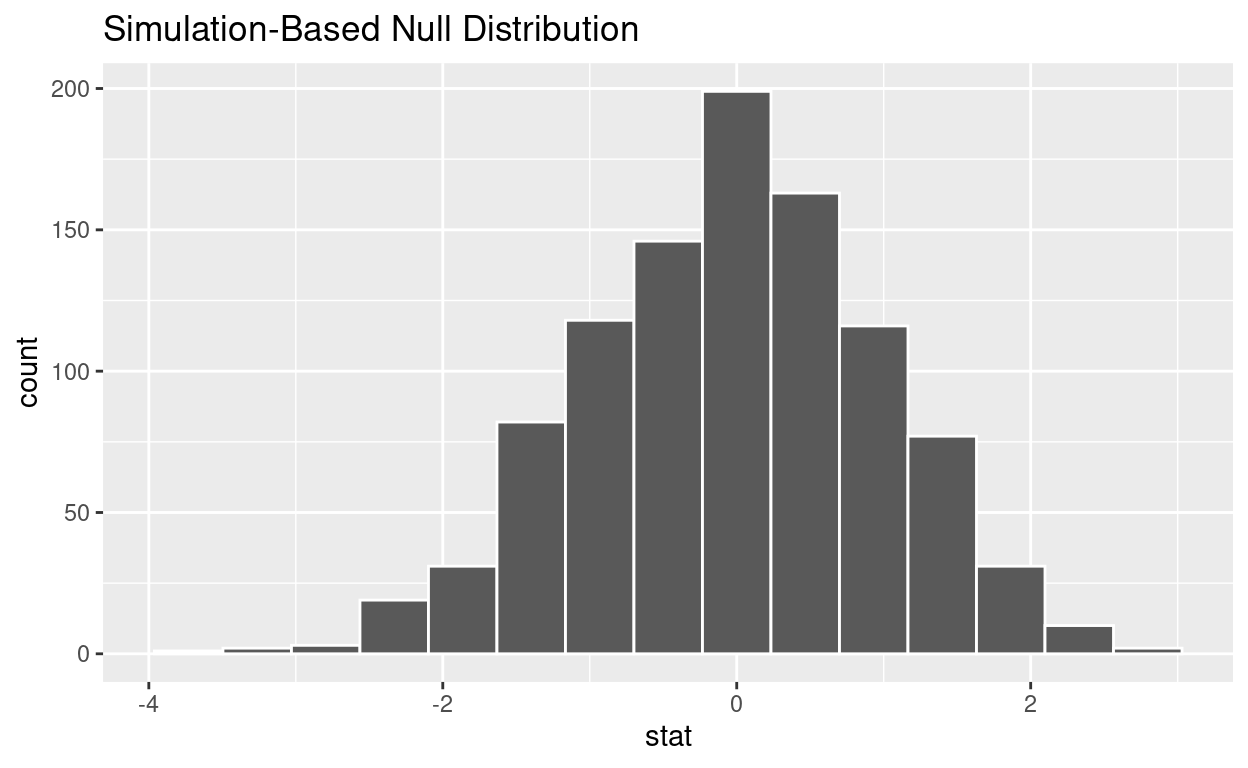`calculate` the statistic from your observed data

• Assign the output `observed_t_2_sample_stat`

• Display `observed_t_2_sample_stat`

``````observed_t_2_sample_stat  <- hr_2 %>%
specify(response = hours, explanatory = gender)  %>%
calculate(stat = "t", order = c("female", "male"))

observed_t_2_sample_stat
``````
``````Response: hours (numeric)
Explanatory: gender (factor)
# A tibble: 1 × 1
stat
<dbl>
1 0.208``````

get_p_value from the simulated null distribution and the observed statistic

``````null_t_distribution  %>%
get_p_value(obs_stat = observed_t_2_sample_stat, direction = "two-sided")
``````
``````# A tibble: 1 × 1
p_value
<dbl>
1   0.822``````

shade_p_value on the simulated null distribution

``````null_t_distribution  %>%
visualize() +
shade_p_value(obs_stat = observed_t_2_sample_stat, direction = "two-sided")
````````````ggsave(filename = "preview.png",
path = here::here("_posts", "2022-05-02-hypothesis-testing"))
``````

Is the p-value < 0.05? no

Does your analysis support the null hypothesis that the true mean number of hours worked by female and male employees was the same? yes

# Question: ANOVA

hr_3_tidy.csv is the name of your data subset

• Read it into and assign to hr_anova

• Note: col_types = “fddfff” defines the column types factor-double-double-factor-factor-factor
``````hr_anova <- read_csv("https://estanny.com/static/week13/data/hr_3_tidy.csv",
col_types = "fddfff")
``````

## Q: Is the average number of hours worked the same for all three status (fired, ok and promoted) ?

use `skim` to summarize the data in `hr_anova` by `status`

``````hr_anova %>%
group_by(status)  %>%
skim()
``````
 Name Piped data Number of rows 500 Number of columns 6 _______________________ Column type frequency: factor 3 numeric 2 ________________________ Group variables status

Variable type: factor

skim_variable status n_missing complete_rate ordered n_unique top_counts
gender promoted 0 1 FALSE 2 fem: 91, mal: 81
gender fired 0 1 FALSE 2 mal: 98, fem: 98
gender ok 0 1 FALSE 2 mal: 68, fem: 64
evaluation promoted 0 1 FALSE 4 goo: 79, ver: 52, fai: 21, bad: 20
evaluation fired 0 1 FALSE 4 bad: 77, fai: 64, ver: 30, goo: 25
evaluation ok 0 1 FALSE 4 fai: 53, bad: 51, goo: 18, ver: 10
salary promoted 0 1 FALSE 6 lev: 42, lev: 37, lev: 36, lev: 28
salary fired 0 1 FALSE 6 lev: 59, lev: 40, lev: 39, lev: 25
salary ok 0 1 FALSE 6 lev: 33, lev: 29, lev: 28, lev: 23

Variable type: numeric

skim_variable status n_missing complete_rate mean sd p0 p25 p50 p75 p100 hist
age promoted 0 1 40.22 11.11 20.1 31.67 41.00 48.82 59.7 ▆▇▇▇▇
age fired 0 1 38.95 11.23 20.0 29.82 38.80 48.75 59.9 ▇▆▇▇▅
age ok 0 1 39.03 11.77 20.0 28.28 38.75 49.92 59.7 ▇▇▆▇▆
hours promoted 0 1 59.29 12.53 35.0 49.90 58.65 70.35 79.9 ▅▆▇▆▇
hours fired 0 1 42.37 9.15 35.0 36.20 39.20 43.80 79.6 ▇▁▁▁▁
hours ok 0 1 47.99 11.55 35.0 37.45 45.75 55.23 75.7 ▇▃▃▂▂
• Employees that were fired worked an average of 42.4 hours per week

• Employees that were ok worked an average of 48.0 hours per week

• Employees that were promoted worked an average of 59.3 hours per week

Use `geom_boxplot` to plot distributions of hours worked by status

``````hr_anova %>%
ggplot(aes(x = status, y = hours)) +
geom_boxplot()
``````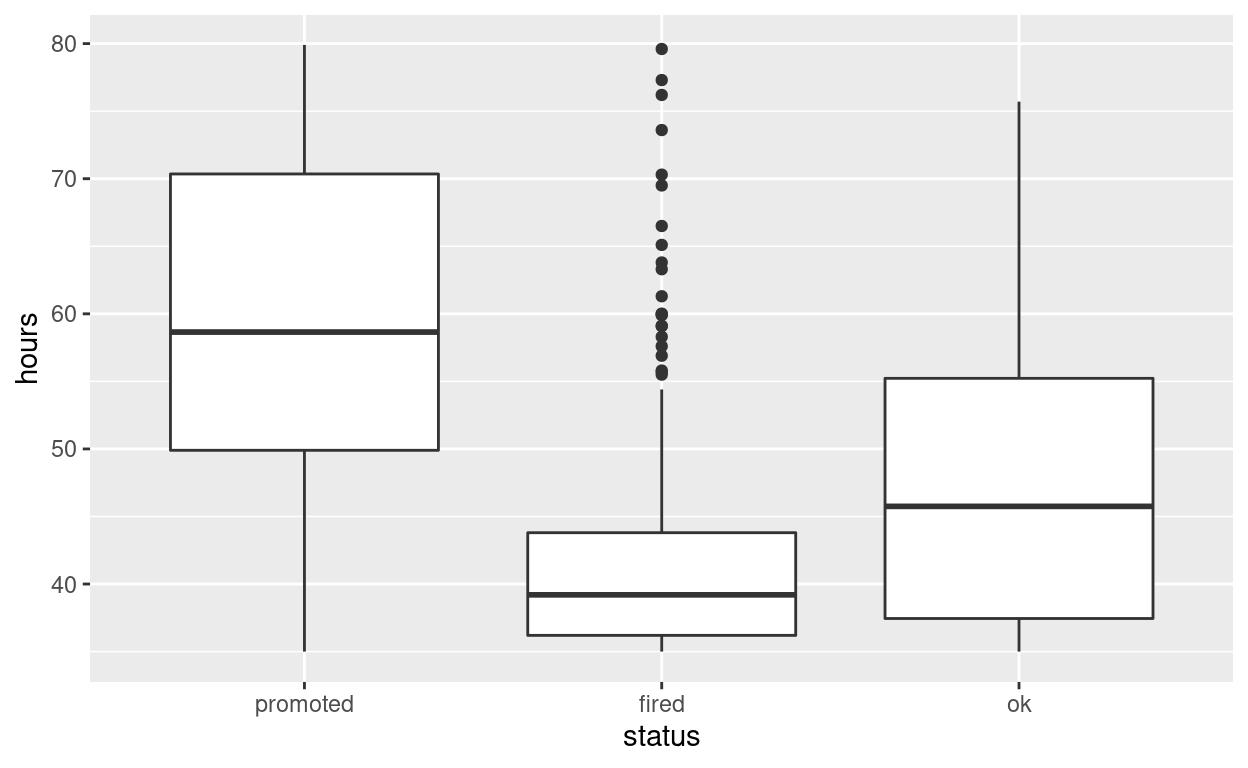`specify` the variables of interest are `hours` and `status`

``````hr_anova %>%
specify(response = hours, explanatory = status)
``````
``````Response: hours (numeric)
Explanatory: status (factor)
# A tibble: 500 × 2
hours status
<dbl> <fct>
1  49.6 promoted
2  39.2 fired
3  63.2 promoted
4  42.2 promoted
5  54.7 promoted
6  54.3 fired
7  37.3 fired
8  45.6 promoted
9  35.1 fired
10  53   promoted
# … with 490 more rows``````

`hypothesize` that the number of hours worked and status are independent

``````hr_anova  %>%
specify(response = hours, explanatory = status)  %>%
hypothesize(null = "independence")
``````
``````Response: hours (numeric)
Explanatory: status (factor)
Null Hypothesis: independence
# A tibble: 500 × 2
hours status
<dbl> <fct>
1  49.6 promoted
2  39.2 fired
3  63.2 promoted
4  42.2 promoted
5  54.7 promoted
6  54.3 fired
7  37.3 fired
8  45.6 promoted
9  35.1 fired
10  53   promoted
# … with 490 more rows``````

`generate` 1000 replicates representing the null hypothesis

``````hr_anova %>%
specify(response = hours, explanatory = status)  %>%
hypothesize(null = "independence")  %>%
generate(reps = 1000, type = "permute")
``````
``````Response: hours (numeric)
Explanatory: status (factor)
Null Hypothesis: independence
# A tibble: 500,000 × 3
# Groups:   replicate [1,000]
hours status   replicate
<dbl> <fct>        <int>
1  38.4 promoted         1
2  41   fired            1
3  66.1 promoted         1
4  46.1 promoted         1
5  65.1 promoted         1
6  43.7 fired            1
7  35.1 fired            1
8  40.9 promoted         1
9  38.4 fired            1
10  67.7 promoted         1
# … with 499,990 more rows``````

The output has 500,000 rows

`calculate` the distribution of statistics from the generated data

• Assign the output `null_distribution_anova`

• Display `null_distribution_anova`

``````null_distribution_anova  <- hr_anova %>%
specify(response = hours, explanatory = status)  %>%
hypothesize(null = "independence")  %>%
generate(reps = 1000, type = "permute")  %>%
calculate(stat = "F")

null_distribution_anova
``````
``````Response: hours (numeric)
Explanatory: status (factor)
Null Hypothesis: independence
# A tibble: 1,000 × 2
replicate   stat
<int>  <dbl>
1         1 0.204
2         2 0.972
3         3 2.16
4         4 1.33
5         5 0.919
6         6 1.14
7         7 1.52
8         8 0.335
9         9 0.500
10        10 0.0298
# … with 990 more rows``````
• `null_distribution_anova` has 1000 F-stats

`visualize` the simulated null distribution

``````visualize(null_distribution_anova)
``````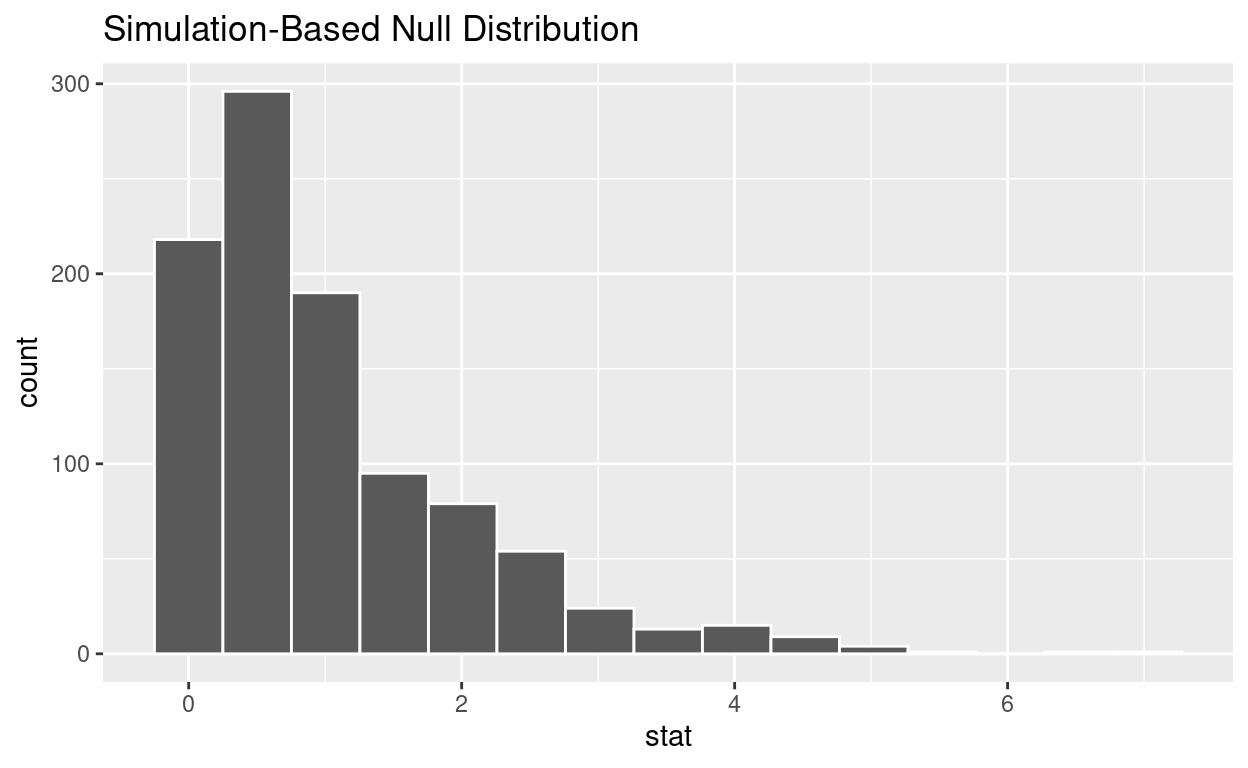`calculate` the statistic from your observed data

• Assign the output `observed_f_sample_stat`

• Display `observed_f_sample_stat`

``````observed_f_sample_stat  <- hr_anova %>%
specify(response = hours, explanatory = status)  %>%
calculate(stat = "F")

observed_f_sample_stat
``````
``````Response: hours (numeric)
Explanatory: status (factor)
# A tibble: 1 × 1
stat
<dbl>
1  110.``````

get_p_value from the simulated null distribution and the observed statistic

``````null_distribution_anova  %>%
get_p_value(obs_stat = observed_f_sample_stat, direction = "greater")
``````
``````# A tibble: 1 × 1
p_value
<dbl>
1       0``````

shade_p_value on the simulated null distribution

``````null_t_distribution  %>%
visualize() +
shade_p_value(obs_stat = observed_f_sample_stat, direction = "greater")
``````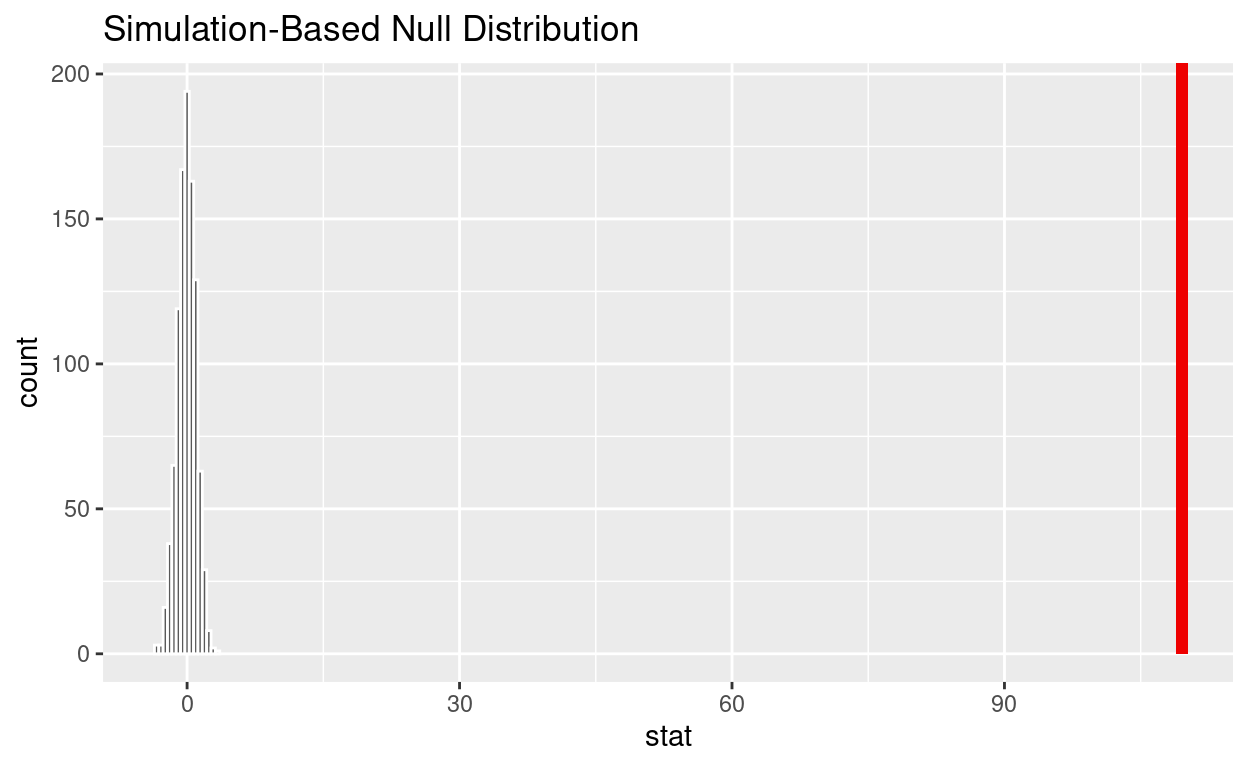• If the p-value < 0.05? yes

• Does your analysis support the null hypothesis that the true means of the number of hours worked for those that were “fired”, “ok” and “promoted” were the same? no